### TL;DR This is a text about differentiation and its geometric...
You can find the entire book here: - [Calculus Made Easy (HTML v...
Silvanus Phillips Thompson was a professor of physics mostly known ...
A visual example of varying slopes at different points of a curve. ...
The tangent to the curve at specific point is parametrized by a lin...
That's funny
Values for the tangent function $\tan$ between $-2\pi$ and $2\pi$. ...
ahhhh
local minimum
In this figure we can see one maximum at $x=1$ and one minimum at $... The simplest case to examine is that of a linear function. The slop... This is a great example where you can visualize the graph for a giv... let's talk about this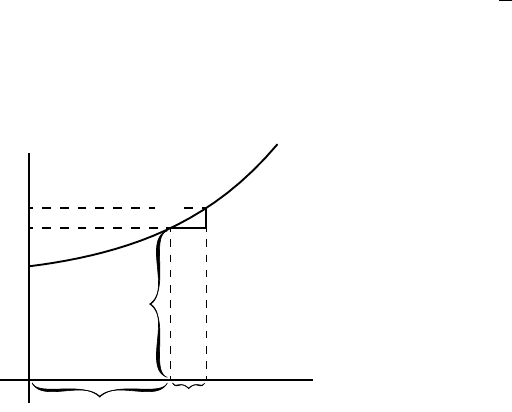CHAPTER X. GEOMETRICAL MEANING OF DIFFERENTIATION. It is useful to consider what geometrical meaning can be given to the dierential coecient. In the ﬁrst place, any function of x,such,forexample,asx 2 ,or p x, or ax + b,canbeplottedasacurve;andnowadayseveryschoolboyis familiar with the process of curve-plotting. dx dy P Q R y x dx O X Y Fig. 7. Let PQR,inFig. 7,beaportionofacurveplottedwithrespect to the axes of coordinates OX and OY .ConsideranypointQ on this curve, where the abscissa of the point is x and its ordinate is y. Now observe how y changes w hen x is varied. If x is made to incr ea se byCALCULUS MADE EASY 76 asmallincrementdx,totheright,itwillbeobservedthaty also (in this particular curve) increases by a small increment dy (because this particular curve happens to be an ascending curve). Then the ratio of dy to dx is a measure of the degree to which the curve is sloping up between the two points Q and T . As a matter of fact, it can be seen on the ﬁgure that the curve between Q and T has m a ny dierent slopes, so that we cann ot very well speak of the slope of the curve between Q and T .If,however,Q and T are so near each other that the small portion QT of the curve is practically straight, then it is true to say that the ratio dy dx is the slope of the curve along QT .ThestraightlineQT produced on either side touches the curve along the portion QT only, and if this portio n is indeﬁnitely small, the straight line will touch the curve at practically one point only, and be therefore a tangent to the curve. This tangent to th e curve has evidently the same slope as QT ,so that dy dx is the slope of the tangent to the curve at the point Q for which the value of dy dx is found. We have seen that the short expression the slope of a curve has no precise meaning, because a curve has so many slopes—in fact, every small portion of a curve has a dierent slope. “The slope of a curve at a point”is,however,aperfectlydenedthing;itistheslopeofavery small portion of the curve situated just at that point; and we have seen that this is the same as “the slope of the tan gent to the curve at that point. Observe that dx is a short step to the right, and dy the correspond- ing short step upwards. These steps must be considered as short as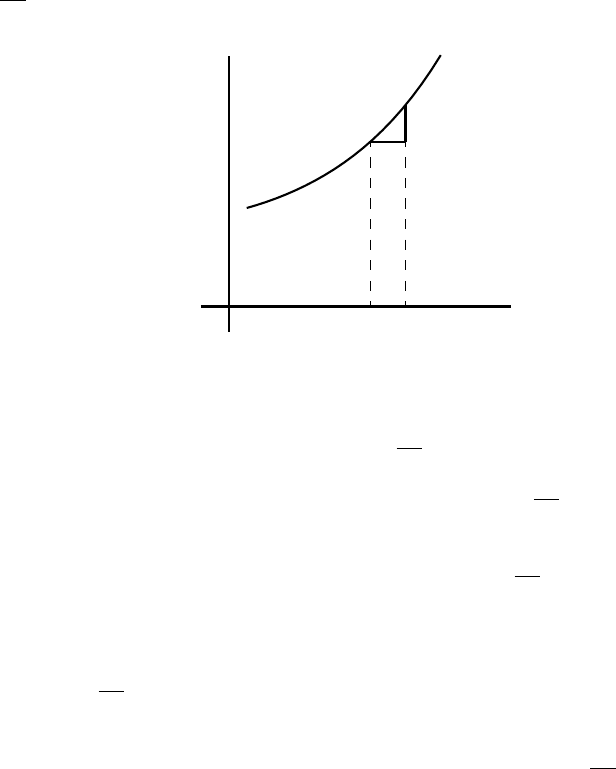MEANING OF DIFF ER ENTI ATION 77 possible—in fact indenitely short,—though in diagrams we have to represent them by bits that are not inﬁni t es i mal l y small, otherwise they could not be seen. We shall hereafter make considerable use of this circumstance that dy dx represents the slope of the curve at any point. dx dy O X Y Fig. 8. If a curve is sloping up at 45 at a particular point, as in Fig. 8, dy and dx will be equal, and the value of dy dx =1. If the curve slopes up steeper t h an 45 (Fig. 9), dy dx will be greater than 1. If the curve slopes up very gently, as i n Fig. 10, dy dx will be a fraction smaller than 1. For a horizontal line, or a horizontal place in a curve, dy =0,and therefore dy dx =0. If a curve slopes downward,asinFig. 11, dy will be a step down, and must t h er efo re be reckoned of negative va l u e; hence dy dx will have negative sign also.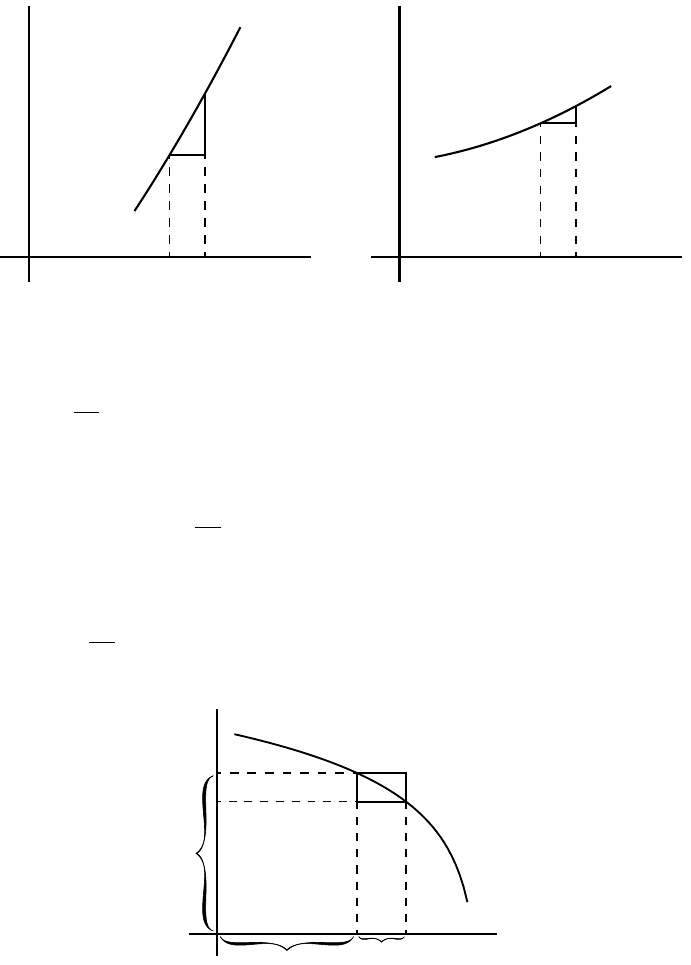CALCULUS MADE EASY 78 dx dy O X Y Fig. 9. dx dy O X Y Fig. 10. If the “curve” happens to be a straight line, like th at in Fig. 12,the value of dy dx will be the same at all points along i t . In other words its slope is constant. If a cur ve is one that turn s mor e u pwards as it goes along to the right, the values of dy dx will b ecom e greater and greater with th e in- creasing steepness, as in Fig. 13. If a curve is one that gets ﬂatter and ﬂatter as it goes along, the values of dy dx will become smaller and smaller as the ﬂatter par t is dx dy Q y x dx O X Y Fig. 11.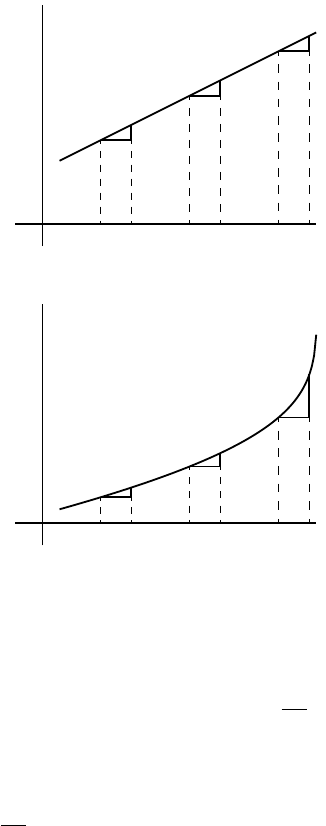MEANING OF DIFF ER ENTI ATION 79 dx dy dx dy dx dy O X Y Fig. 12. dx dy dx dy dx dy O X Y Fig. 13. reached, as in Fig. 14. If a curve ﬁrst descends, and then goes u p agai n , as in Fig. 15, presenting a concavity upwards, then clearly dy dx will ﬁrst be negative, with diminishing values as the curve ﬂattens, then will be zero at the point where the bottom of the trough of the curve is reached; and from this point onward dy dx will have positive values that go on incr easin g. In such a case y is said to pass by a minimum.Theminimumvalueofy is not necessarily the smallest value of y,itisthatvalueofy corresponding to the bottom of the trough; for instance, in Fig. 28 (p. 99), the value of y corr espondi n g to the bottom of the trough is 1, while y takes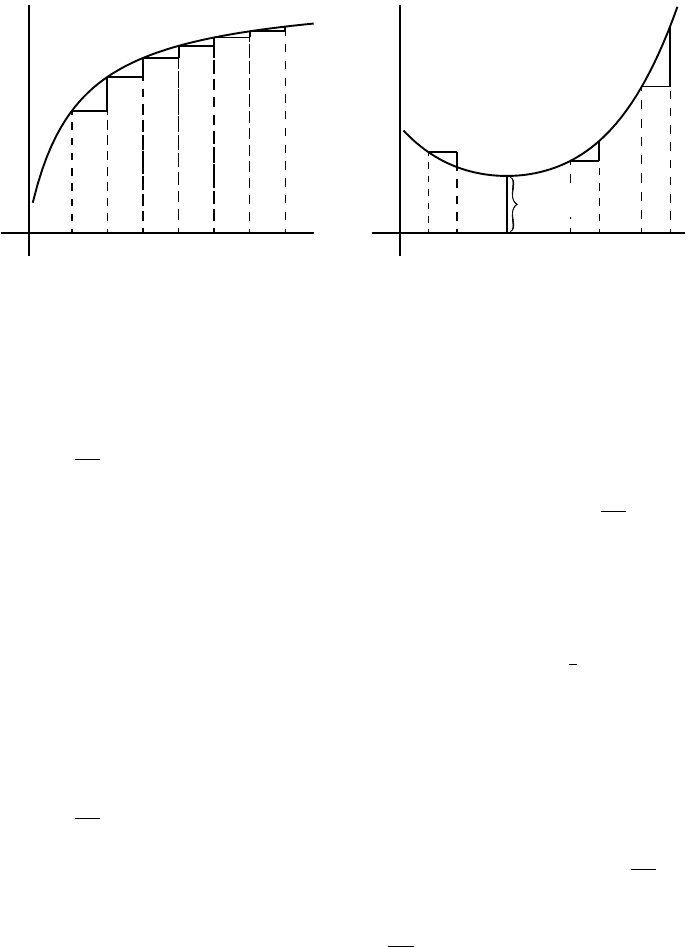CALCULUS MADE EASY 80 O X Y Fig. 14. O X Y y min. Fig. 15. elsewhere values which are smaller than this. The characteristic of a minimum is that y must increase on either side of it. N.B.—For the particular value of x that makes y a minimum,the value of dy dx =0. If a curve ﬁrst ascends and then descends, the values of dy dx will be positive at rst; then zero, as the summit is reached; then negative, as the curve slopes downwards, as in Fig . 16.Inthiscasey is said to pass by a maximum,butthemaximumvalueofy is not necessarily the greatest value of y.InFig. 28,themaximumofy is 2 1 3 ,butthisis by no means the greatest value y can have at some other point of the curve. N.B.—For the particular value of x that makes y a maximum,the value of dy dx =0. If a curve has the peculiar form of Fig. 17,thevaluesof dy dx will always be positive; but there will be one particular place where t h e slope is least steep, where the value of dy dx will be a minimum; that is, less than it is at any other part of the curve.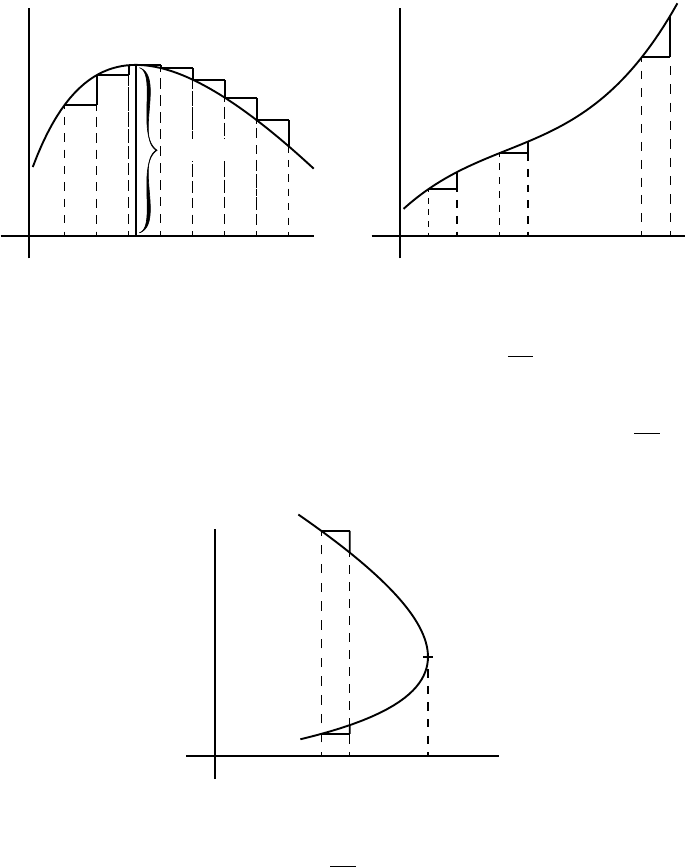MEANING OF DIFF ER ENTI ATION 81 O X Y y max. Fig. 16. O X Y Fig. 17. If a curve has the form of Fi g . 18,thevalueof dy dx will be negative in the upper part, and positive in the lower part; while at the nose of the curve where it becomes actually perpendicular, the value of dy dx will be inﬁnitely great. dx dx dy dy Q O X Y Fig. 18. Now that we understand that dy dx measures the steepness of a curve at any point, let us turn to some of the equations which we have already learned how to dierentiate.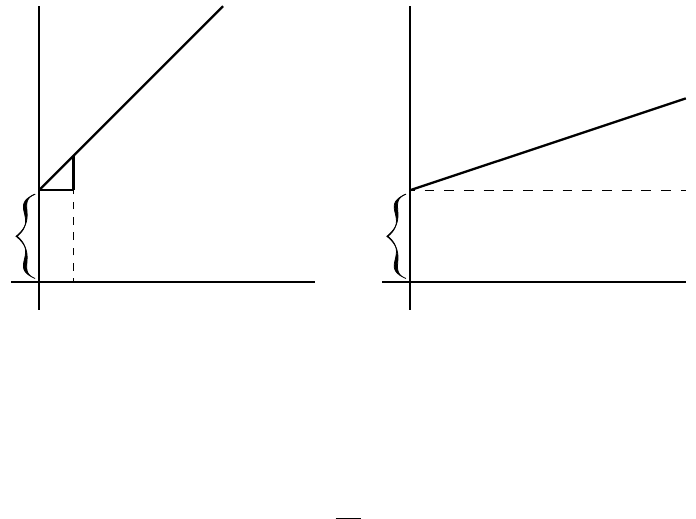CALCULUS MADE EASY 82 (1) As the simplest case take this: y = x + b. It is plotted out in Fig. 19,usingequalscalesforx and y.Ifweput x =0,thenthecorrespondingordinatewillbey = b;thatistosay,the “curve” crosses the y-axi s at the height b.Fromhereitascendsat45 ; dx dy b O X Y Fig. 19. b O X Y Fig. 20. for whatever values we give to x to the right, we have an equal y to ascend. The line has a gradient of 1 in 1. Now dierentiate y = x + b,bytheruleswehavealreadylearned (pp. 21 and 25 ante), and we get dy dx =1. The slope of the l i n e is such t h at for every little step dx to the right, we go an equal little step dy upward. And this slope is constant—always the same slope. (2) Take another case: y = ax + b.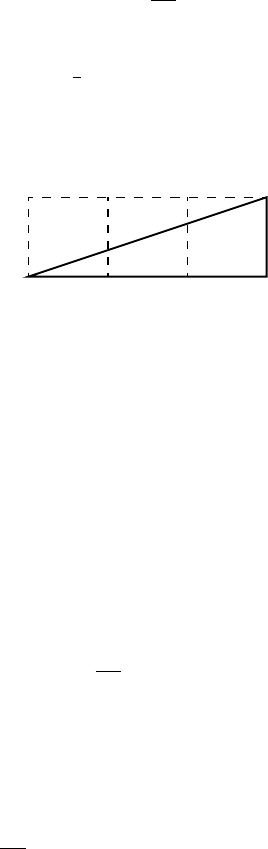MEANING OF DIFF ER ENTI ATION 83 We know that this curve, like the preceding one, will start from a height b on the y-axis. But before we draw the curve, let us ﬁnd i ts slope by dierentiating; which gives dy dx = a.Theslopewillbeconstant, at an angle, the tangent of which is here called a.Letusassigntoa some numerical value—say 1 3 . Then we must give it such a slope that it ascends 1 in 3; or dx will be 3 times as great as dy;asmagniedin Fig. 21.So,drawthelineinFi g. 20 at this slope. Fig. 21. (3) Now for a slightly harder case. Let y = ax 2 + b. Again the curve will start on the y-axis at a height b above the origin. Now dierentiat e. [If you have forgotte n , turn back to p. 25;or, rather, don’t turn back, but think out the dierentiation.] dy dx =2ax. This shows that the steepness will not be constant: it increases as x increases. At the starting point P ,wherex =0,thecurve(Fig. 22) has no steepness—that is, it is level. On the left o f the origin, where x has negative values, dy dx will also have negative valu es, or will descend from left to right, as in the Figure.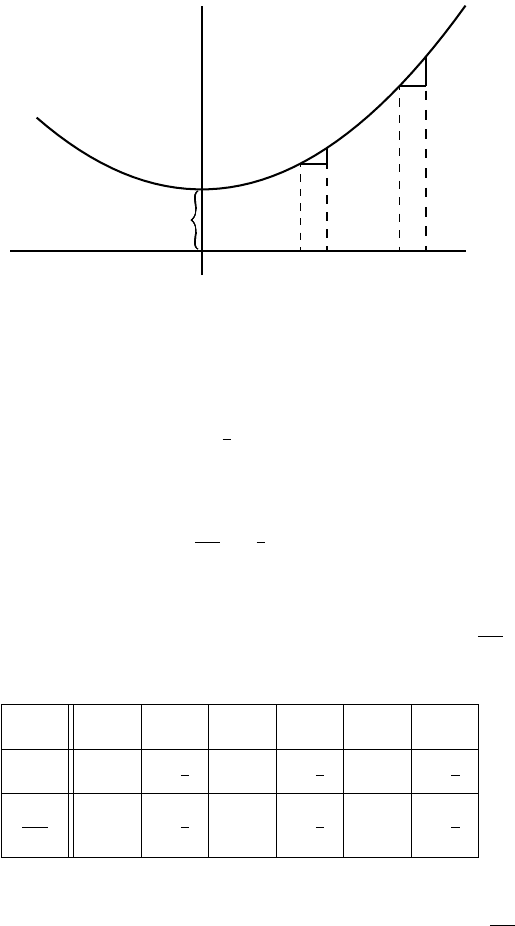CALCULUS MADE EASY 84 P Q R b O X Y Fig. 22. Let us illustrate this by working out a particular instance. Taking the equation y = 1 4 x 2 +3, and dierentiating it, we get dy dx = 1 2 x. Now assign a few successive values, say from 0 to 5, to x;andcalculate the corresponding values of y by the rst equation; and of dy dx from the second equat i on . Tab u l at i ng results, we have: x 0 1 2 3 4 5 y 3 3 1 4 4 5 1 4 7 9 1 4 dy dx 0 1 2 1 1 1 2 2 2 1 2 Then plot them out in two curves, Figs. 23 and 24,inFig. 23 plotting the values of y against those of x and in Fig. 24 those of dy dx against those of x.Foranyassignedvalueofx,theheight of the ordinate in the second curve is proportional to the slope of the ﬁrst curve.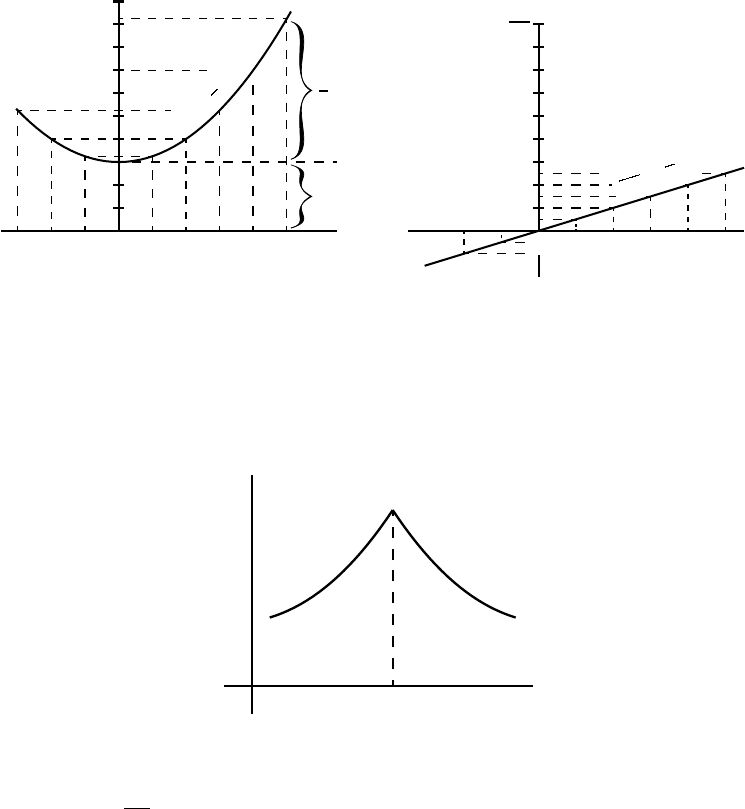MEANING OF DIFF ER ENTI ATION 85 3 2 10 1 2 3 4 5 5 6 7 8 9 x y b 1 4 x 2 y = 1 4 x 2 +3 Fig. 23. 3 2 1 12345 1 2 3 4 5 x dy dx 0 dy dx = 1 2 x Fig. 24. If a curve comes to a sudden cusp, as in Fig. 25,theslopeatthat point suddenly changes from a slope upward to a slope downward. In O X Y Fig. 25. that case dy dx will clearly undergo an abrupt change from a positive to anegativevalue. The following examples show further applications of the prin cip l es just explain ed .CALCULUS MADE EASY 86 (4) Find the slope of the tangent to the curve y = 1 2x +3, at the point where x = 1. Find the angle which this tangent makes with the curve y =2x 2 +2. The slope of the tangent is the slope of t h e curve at the point where they touch one another (see p. 76); that is, it is the dy dx of the curve for that point. Here dy dx = 1 2x 2 and for x = 1, dy dx = 1 2 ,whichisthe slope of the tangent and of the curve at that point. The tangent, being astraightline,hasforequationy = ax + b,anditsslopeis dy dx = a, hence a = 1 2 . Also if x = 1, y = 1 2(1) +3 = 2 1 2 ;andasthe tangent passes by this point, the coordinates of the point must satisfy the equation of the tangent, namely y = 1 2 x + b, so that 2 1 2 = 1 2 (1) + b and b = 2; the eq u a t i on of the tangent is therefore y = 1 2 x +2. Now, when two curves meet, the intersection being a point com- mon to both curves, its co ordinates must satisfy the equation of each one of the two curves; that is, it must be a solution of the system of simultaneous equations formed by coupling together the equations of the curves. Here the curves meet o n e another at points given by the solution of 8 < : y =2x 2 +2, y = 1 2 x +2 or 2x 2 +2= 1 2 x +2;MEANING OF DIFF ER ENTI ATION 87 that is, x(2x + 1 2 )=0. This equation has for its solutions x =0andx = 1 4 .Theslopeof the curve y =2x 2 +2atanypointis dy dx =4x. For the point where x = 0, this slope is zero; the curve is h or i zo ntal. For the p oint where x = 1 4 , dy dx = 1; hence the curve at that point slopes downwards to the right at such an angle with the horizontal that tan = 1; that is, at 45 to the horizontal. The slope of the straight line is 1 2 ;thatis,itslopesdownwardsto the right and makes with the horizontal an angle such that t an = 1 2 ; that is, an angle of 26 34 0 .Itfollowsthatattherstpointthecurve cuts the straight line at an angle of 26 34 0 ,whileattheseconditcuts it at an angle of 45 26 34 0 =18 26 0 . (5) A straight line is to be drawn, through a point whose coordinates are x =2,y = 1, as tangent to the curve y = x 2 5x + 6. Find the coordinates of the p oi nt of contact. The sl ope of the tangent must be the same as the dy dx of the curve; that is, 2x 5. The equation of the straight line is y = ax+b,andasitissatisedfor the values x =2,y = 1, then 1=a2+b;also,its dy dx = a =2x5. The x and the y of the point of contact must also satisfy both the equation o f the tangent and the equation of the curve.CALCULUS MADE EASY 88 We have then y = x 2 5x +6, (i) y = ax + b, (ii) 8 > > > > > > < > > > > > > : 1=2a + b, (iii) a =2x 5, (iv) four equation s in a, b, x, y. Equations (i ) and (ii) give x 2 5x +6=ax + b. Replacing a and b by their v alue in this, we get x 2 5x +6=(2x 5)x 1 2(2x 5), which simpli ﬁ es to x 2 4x +3=0,thesolutionsofwhich are: x =3 and x =1. Replacingin(i),wegety =0andy =2respectively;the two points of contact are then x =1,y =2,andx =3,y =0. Note.—In all exercises dealing with curves, students will ﬁnd it ex- tremely instructive to verify the deductions obtained by actually plot- ting the curves. Exercises VII I . (See page 256 for Answers.) (1) Plot the c urve y = 3 4 x 2 5, using a scale of millimetres. Measure at points corresponding to dierent values of x,theangleofitsslope. Find, by dierentiating the equation, the expression for slope; and see, from a Table of Natural Tangents, whether this agrees with the measured angle.MEANING OF DIFF ER ENTI ATION 89 (2) Find what will be the slope of the curve y =0.12x 3 2, at the particular point that has as abscissa x =2. (3) If y =(x a)(x b) , show that at the particular point of the curve wh er e dy dx =0,x will have the value 1 2 (a + b). (4) Find the dy dx of the equation y = x 3 +3x;andcalculatethe numerical values of dy dx for the points corresponding to x =0,x = 1 2 , x =1,x =2. (5) In the curve to which the equation is x 2 +y 2 =4,ndthevalues of x at those points where the slope = 1. (6) Find the slope, at any point, of the curve whose equation is x 2 3 2 + y 2 2 2 =1;andgivethenumericalvalueoftheslopeattheplace where x =0,andatthatwherex =1. (7) The equation of a tangent to the curve y =52x +0.5x 3 ,being of the form y = mx + n,wherem and n are constants , ﬁnd the value of m and n if the point where the tangent touches the curve has x =2 for abscissa. (8) At what angle do the two curves y =3.5x 2 +2 and y = x 2 5x +9.5 cut one another? (9) Tangents to the curve y = ± p 25 x 2 are drawn at points for which x =3andx = 4. Fin d the coordinates of the point of intersection of the tangents and their mutual inclination. CALCULUS MADE EASY 90 (10) Astraightliney =2x b touches a curve y =3x 2 +2at one point. What are the coordinat es of the point of contact, and what is the value of b? The simplest case to examine is that of a linear function. The slope$a$of a linear function is a constant. In this first example the author choose the easiest of all$a=1$. This is a great example where you can visualize the graph for a given function$y=f(x)$on Figure 23 and the graph for it's derivative$\frac{dy}{dx}=f^{\prime}(x)$of that function Figure 24. local minimum The tangent to the curve at specific point is parametrized by a linear function as follows$y = ax+b$where a is the slope of that curve and is computed as follows: \begin{eqnarray*} a&=&{\frac {{\text{change in }}y}{{\text{change in }}x}}\\ &=&{\frac {\Delta y}{\Delta x}}\\ &=&{\tan{\theta}} \end{eqnarray*} ![differentiation](https://upload.wikimedia.org/wikipedia/commons/thumb/c/c1/Wiki_slope_in_2d.svg/562px-Wiki_slope_in_2d.svg.png) *Figure: Slope of a linear function.* You can find the entire book here: - [Calculus Made Easy (HTML version)](https://calculusmadeeasy.org) - [The Project Gutenberg EBook of Calculus Made Easy, by Silvanus Thompson (PDF version)](http://www.gutenberg.org/files/33283/33283-pdf.pdf) Values for the tangent function$\tan$between$-2\pi$and$2\pi$. Note that for an angle$\theta = 45^{\circ} = \frac{\pi}{4}$:$\tan{(45^{\circ})}= \tan{(\frac{\pi}{4})} = 1$!["tan plot"](https://i.imgur.com/BoRR8gT.png) A visual example of varying slopes at different points of a curve. Here the values for the slope are given by$f^{\prime}$at different points$A$for the function$f(x)=x\sin{(x^2)}+1$. !["slopes"](https://upload.wikimedia.org/wikipedia/commons/2/2d/Tangent_function_animation.gif) let's talk about this ahhhh That's funny In this figure we can see one maximum at$x=1$and one minimum at$x=3$. The slope **a** of the tangent to the curve at those points is 0, i.e.$a=\frac{dy}{dx} = 0$. To the left of a minimum we have that$a<0$and to the right$a>0$. Inversely for a maximum we have that to the left of it the slope is$a>0$and to the right$a<0\$. !["figure convex"](https://i.imgur.com/ExouW1t.png) Silvanus Phillips Thompson was a professor of physics mostly known for his work as an electrical engineer. He was elected to the Royal Society 1891. Thompson was also an author and he published his most famous book **Calculus Made Easy** in 1910. This book is one of the greatest introductions to the fundamentals of infinitesimal calculus, and is still in print today. Learn more about the author here: - [Silvanus P. Thompson 1851-1916 Professor of Physics - Electrical Engineer](http://www.engineerswalk.co.uk/st_walk.html) - [Silvanus P. Thompson](https://en.wikipedia.org/wiki/Silvanus_P._Thompson) !["spt"](https://upload.wikimedia.org/wikipedia/commons/4/4a/Thompson_Silvanus_mature.jpg) ### TL;DR This is a text about differentiation and its geometrical meaning from the book "Calculus Made Easy" originally published in 1910 by Silvanus P. Thompson. It constitutes one of the greatest and most elegant introductions to the topic of calculus. It's worth reading even if you know math really well because it will help you understand how to teach complexity without any added fluff or overt technicality to others. It's a fascinating book. Excerpt from its Prologue: > ***Considering how many fools can calculate, it is surprising that it should be thought either a difficult or a tedious task for any other fool to learn how to master the same tricks.*** >*** Some calculus tricks are quite easy. Some are enormously difficult. The fools who write the textbooks of advanced mathematics - and they are mostly clever fools - seldom take the trouble to show you how easy the easy calculations are. On the contrary, they seem to desire to impress you with their tremendous cleverness by going about it in the most difficult way.*** >*** Being myself a remarkably stupid fellow, I have had to unteach myself the difficulties, and now beg to present to my fellow fools the parts that are not hard. Master these thoroughly, and the rest will follow. What one fool can do, another can.***